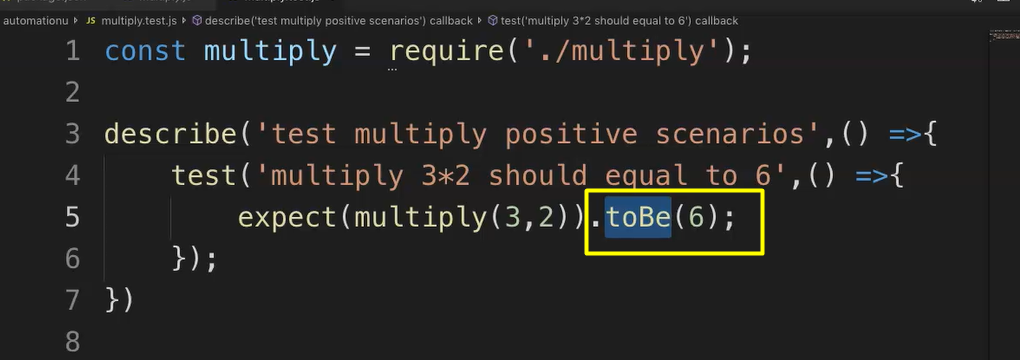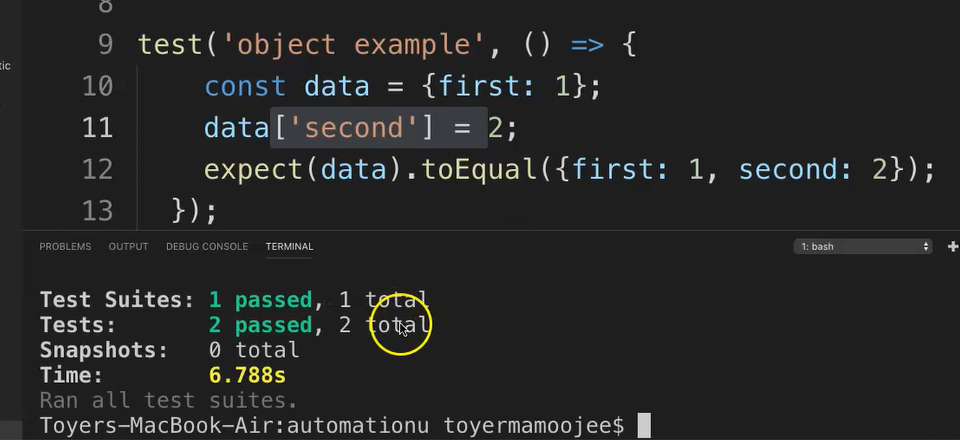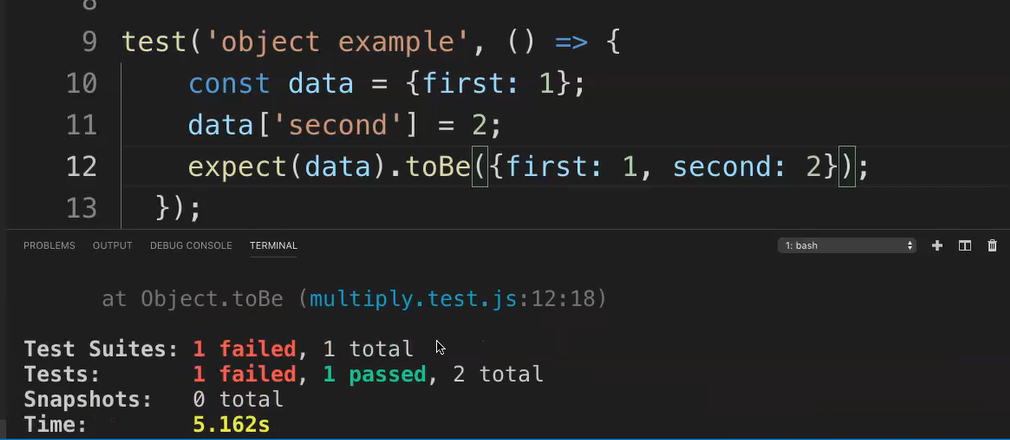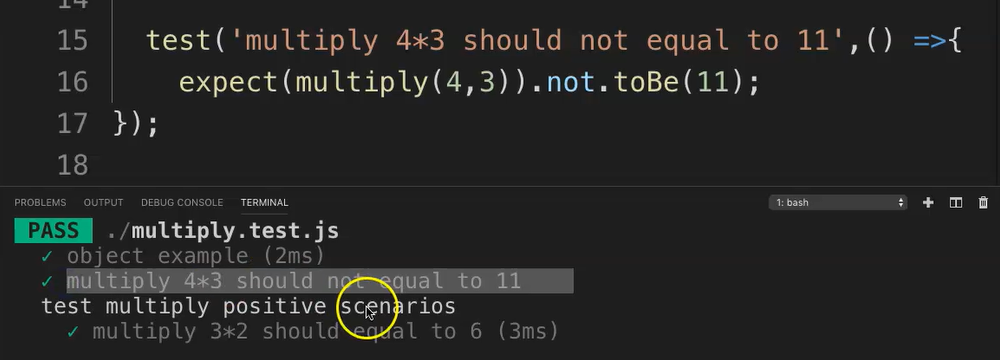Transcripted Summary

In our previous lesson, we saw an example of a matcher, but I deliberately did not go into the detail of it, due to this particular focused lesson.

### # What are matchers?

Typically, matchers are methods that let you test your values.

Ideally, you will always use a matcher in conjunction with a `.expect`.

The two most common matches are `toBe` and `toEqual`.

• `toBe()` uses strict equality.
• `toEqual()` tests the value of an object.

In our previous lesson in the example that we used it would really make sense to use `toBe` here:As we are testing for values and they are being calculated.

However, the moment we get something like this (and I am going to be pasting another test in here):

### # multiply.test.js – comparing `toBe` and `toEqual`

``````const multiply = require('./multiply');

describe('test multiply positive scenarios',() =>{
test('multiply 3*2 should equal to 6',() =>{
expect(multiply(3,2)).toBe(6);
});
})

test('object example', () => {
const data = {first: 1};
data['second'] = 2;
expect(data).toEqual({first: 1, second: 2});
});
``````

If you look at this 2nd test, we're basically adding an object `first` and then adding a `second` to it with that value.

And then expecting the data to equal `first` and `second` [`expect(data).toEqual({first: 1, second: 2})`].

Now, if you run this, it will run both the tests.

The point here is just to show `toEqual` versus `toBe`.

The tests now run…This now passes, as you can see.

Let's now change `toEqual` to `toBe`

``````test(‘object example', () => {
const data = {first: 1};
data['second'] = 2;
expect(data).toBe({first: 1, second: 2});
});
``````

Let's save this.

And now let's execute.You can now see the failure because we cannot use `toBe`, which is reserved for value checking.

### # Finally, we'll look at another quick common matcher, and that is to use a `not`.

Let's take the previous test and add a `not` before the `toBe`[`not.toBe`], then copy the previous tests and then paste it here.

Maybe let's just change the value to “4*3 should not equal to 11”. Then let's change those inputs, 4 and 3.

Okay, and now we add `not`.

``````test('multiply 4*3 should not equal to 11',() =>{
expect(multiply(4,3)).not.toBe(11);
});
``````

Let's fix this other test also, so it doesn't fail this time.

Let's see what happens now.

There we go.It passes.

That's it for the section on common matchers in Jest.